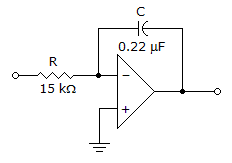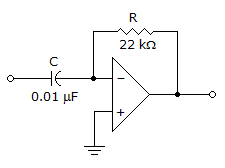# Electronic Devices - Basic Op-Amp Circuits

Exercise : Basic Op-Amp Circuits - General Questions
1.
A Schmitt trigger is
a comparator with only one trigger point.
a comparator with hysteresis.
a comparator with three trigger points.
none of the above.
Explanation:
No answer description is available. Let's discuss.

2.
Refer to the given figure. This circuit is known asa noninverting amplifier.
a differentiator.
an integrator.
a summing amplifier.
Explanation:
No answer description is available. Let's discuss.

3.
The output of a Schmitt trigger is a
pulse waveform.
sawtooth waveform.
sinusoidal waveform.
triangle waveform.
Explanation:
No answer description is available. Let's discuss.

4.
Refer to the given figure. This circuit is known asa noninverting amplifier.
a differentiator.
an integrator.
a summing amplifier.
Explanation:
No answer description is available. Let's discuss.

5.
Refer to the given figure. A square-wave input is applied to this amplifier. The output voltage is most likely to bea square wave.
a triangle wave.
a sine wave.
no output.